### 2.3.2.4 Statistical Averages

Instead of the statistical averages found in eqns. (2.73) to (2.75) and eqns. (2.90) to (2.92) the following state variables are commonly used:
• Densities: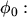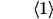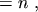(2.93)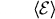(2.94)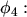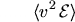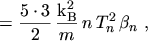(2.95)

• Fluxes: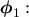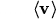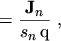(2.96)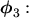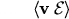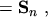(2.97)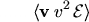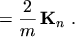(2.98)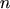is the electron concentration,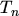is the electron temperature, and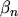is the kurtosis. Eqn. (2.93) represents the normalization of the distribution function chosen in this work. Formulation (2.94) will be explained in more detail in Section 2.3.3.2 on page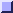. Eqn. (2.95) will be justified in Section 2.3.3.5 on page.

Eqn. (2.96) is defined in analogy to the drift current eqn. (2.21). Eqns. (2.97) and (2.98) are reasonable extensions of the flux term to energy and kurtosis.

Introducing these new state variables leads to the final form of the transport equations: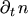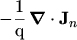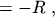(2.99)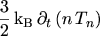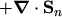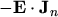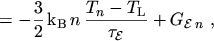(2.100)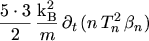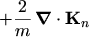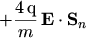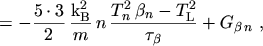(2.101)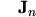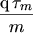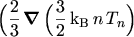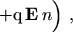(2.102)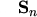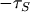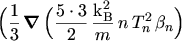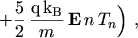(2.103)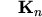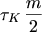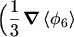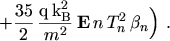(2.104)

M. Gritsch: Numerical Modeling of Silicon-on-Insulator MOSFETs PDF